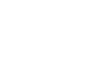Which type of questions do they ask in the AMU BSc entrance exam? - letsdiskussdeepak pandit

@letsuser | Posted on | Education

Which type of questions do they ask in the AMU BSc entrance exam?

0
0

Student | Posted on

Q - Which type of questions do they ask in the AMU BSc Entrance exam?There is a very generalised concept of any undergraduate admission examination. The basics include the clarity in the concepts of Science and Maths. Moreover above average English abilities are also required.
There are four sections that consist of 25 Marks each. Be strong in English, Physics and Chemistry and be very strong in your chosen optional either Mathematics or Biology.
Taking each section at a time :-
English - Comprehension based questions. Though the question will be multiple choice but there are chances that some answers will be very close. You will have to understand the paragraph and then answer the questions. Idioms and phrases, synonyms and antonyms are very much important as they can be directly asked. Be strong in your grammar since you may be asked to find the errors in certain questions. And lastly subject verb relationship is must to prepare.

Physics - Be prepared for logic based questions. You will have to be thorough and crystal clear with all the concepts of class 11th and 12th. Read the NCERTs properly. Mechanics, Electrostatics, Optics and Magnetism are must cover topic along with the numericals. Do not rote-learn any formula since the questions will not be directly based at all. Some free body diagrams and also some whether if questions can be asked. Be prepared for them.

Chemistry - Shape, structure, and chemical formula is very important in Inorganic chemistry as they can be asked directly. Direct numerical based question from Physical Chemistry, from mass number, chemical equilibrium, rate chemistry and Thermodynamics. If you understand thermodynamics once thoroughly you will cover around five questions from both Physics and Chemistry. Revise mechanisms in Organic chemistry and learn all the name reactions as they will be asked directly and easy to choose from the options.

Mathematics (choice based) - The subject requires a lot of practice. Limits, continuity, differentiation, integration are a must. Once answer is sure go for it. Revise all formulas and apply them in questions. Probability and Statistics also will be there. 3D geometry, co-ordinate, matrices and determinants are necessary. The key is practice,practice and practice.

Biology (Choice based) - Each and every diagram from NCERT of class 11th and 12th is must and after that flowcharts and summary of each chapter. The questions asked will be difficult and above average. Attend the ones you are very well confirmed about.
If you prepare by reading each and every chapter by reading the lines and only refer to one side book, the concepts will be better understood and also there will be less confusion.
Assertion- Reasoning questions may be asked.

The Maximum amount of time would be of two hours to attend the paper. Divide the time accordingly and also be prepared to rush through at the end

0
0